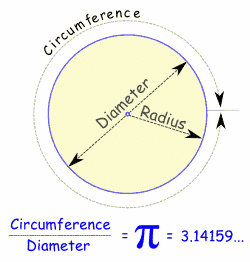# what is pi

Hi!
imbaai...  correctly answers the question.

Good effort!
Your answers are really helpful to all the users of this community.
Keep writing!!!

Cheers!

• -12

# PiPi (the symbol is the Greek letter π) is:The ratio of the Circumference to the Diameter of a Circle.In other words, if you measure the circumference, and then divide by the diameter of the circle you get the number πIt is approximately equal to:3.14159265358979323846…The digits go on and on with no pattern. In fact, pi has been calculated to over one million decimal places and still there is no pattern.

## Approximation

A quick and easy approximation to Pi is 22/7

22/7 = 3.1428571...

But as you can see, 22/7 is not exactly right. In fact Pi is not equal to the ratio of any two numbers, which makes it an irrational number.

• 13
What are you looking for?